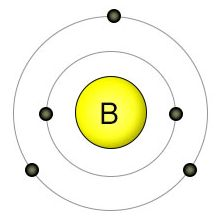# How many electrons does boron have?

Oct 13, 2016

Boron atomic number 5 has five electrons in its ground state.
Commonly Boron will lose 3 electrons leaving 2 electrons in its most common ionic form.

#### Explanation:

The atomic number gives the number of protons. Protons which have a positive charge are balanced by an equal number of electrons in a neutral atom.

Boron number 5 has five protons and therefore as a neutral atom also has five electrons.

Boron has an electron configuration of $1 {s}^{2} 2 {s}^{2} 2 {p}^{1}$

The most stable electron configuration for Boron is

$1 {s}^{2}$ + 3 charge. By losing three electrons Boron can achieve the stable electron structure of Helium

Nov 3, 2016

Boron has five electrons.

#### Explanation:

Boron is element number 5 in the Periodic Table.That means it contains five protons and five electrons.

They are arranged with two electrons in the first energy shell and three in the second.A chemist would say that the electron configuration of boron is

$\text{1s"^2 "2s"^2 "2p}$